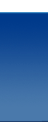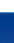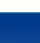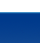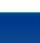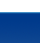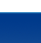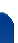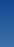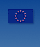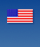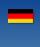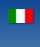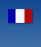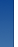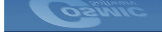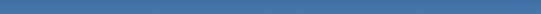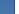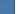Cosmic Software Frequently Asked Questions The results of an expression evaluation seem to be truncated or incorrect. When do I need to add a cast to a C expression? There are many ANSI rules that may require a cast to achieve the expected results, however we find that the most often misunderstood rules are the "Usual Unary and Binary Conversions" rules where types are converted or promoted in expressions. The following descriptions and examples are not intended to be a comprehensive discussion on this topic. It is only intended to describe simply common problems that have been reported to Cosmic's technical support department. Please consult an ANSI reference manual: such as Harbison and Steele's "C A Reference Manual". Cosmic tech support has recorded two common misconceptions which I will illustrate here. In general ANSI rules dictate a base type of "int" which is a 16 bit data type in all Cosmic Software 8 and 16 bit compilers. All expressions are evaluated using this base type (signed int) when all of the arguments of the expression can be represented by a signed integer. The rule applies to the expression arguments and makes no assumption or promotion based on the result of the expression or sub expression which can exceed the representation of an int and cause undesirable results. Some users have incorrectly assumed that the type of an Lvalue affects the promotion rules and subsequently the results of an expression. The type of an lvalue which is the object to the left side of the = operator in an expression has NO affect on an expression except when the result is stored where truncation, sign extension or conversion may occur. An exception to this rule is allowed in the case of a char lvalue set equal to an expression with only char arguments and no promotion casts. The expression may be evaluated at char precision if and only if the result would be identical in all cases if the expression evaluation was promoted to int. Literal constants are assumed to be of type int unless a cast is applied. Example 1 ```unsigned char ch1, ch2; int in1, in2; unsigned int uin1, uin2; long lg1, lg2; unsigned long ulg1, ulg2; ulg1 = (uin1 * uin2) /ch1; ``` According to ANSI rules, the expression above is evaluated using unsigned integer expressions. However, the result of the expression could be larger than an integer so the expected results could be truncated. Adding a cast to promote one of the arguments to unsigned long will make sure the whole expression is evaluated with unsigned long precision and no truncation will occur. e.g. ulg1 = ((unsigned long)uin1 * uin2) /ch1; Note the type of lvalue (ulg1) has no affect on the expression evaluation. Example 2 ```uin1 = 20000; uin2 = 20000; lg = uin1 * uin2; ``` The result of the expression is 33,792 (HC12) when the desired result should be 400,000,000 The expression requires a cast to promote one of the arguments to a long. i.e. ```lg = (long)uin1 * uin2; ``` With the cast the result of the expression is the desired result of 400,000,000 Example3 ```uch1 = 125; uch2 = 220; in1 = uch1 * uch2; ``` The result of the expression above is 27,500 as expected as char types are promoted to int for expression evaluation. No cast is needed.